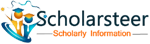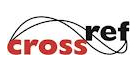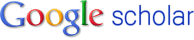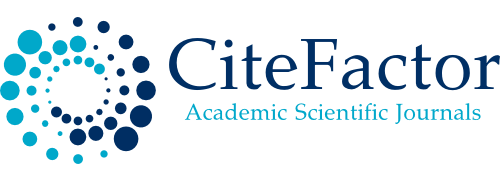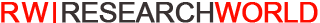#### Call For Paper

Physics

• Physical science
• Physics
• Mathematical physics
• Stochastic analysis
• Stochastic processes
• Development of mathematical methods
• Numerical methods
• Analytic methods
• Microlocal methods
• Mathematical methods for application to problems in physics
• Global analysis
• Formulation of physical theories
• Applied mathematics
• Classical mechanics
• Partial differential equations
• Quantum theory
• Relativity and quantum relativistic theories
• Statistical mechanics
• Prominent mathematical physicists
• Before Newton
• Newtonian and post-Newtonian
• Relativistic
• Quantum
• Theoretical physics
• Experimental physics
• Scientific method
• Relationship between mathematics and physics
• Theory and experiment
• Scope and aims
• Research fields
• Particle physics
• Atomic, molecular and optical physics
• Condensed matter physics
• Astrophysics
• Current research
• Space and time
• Energy and force
• New technologies

## Indexed In